Need help with rubiks-cube?
Click the “chat” button below for chat support from the developer who created it, or find similar developers for support.chrishunt124 Stars21 ForksMIT License141 Commits1 Opened issues#### Description

Solve your Rubik's Cube with an easy two-cycle solution!
?

#### Need anything else?#### Contributors list# 25,739
Ruby
iterm2
strateg...
puzzle-...
99 commits# 776,277
Ruby
1 commit

# Rubik's Cube

Solve your Rubik's Cube with an easy two-cycle solution

## Description

Can you solve the Rubik's Cube? Do you want to learn how? GREAT!

We will teach you a very simple two-cycle solution for solving the Rubik's Cube. It's so simple that I used this exact solution to solve the cube blindfolded in the 2005 Rubik's World Championship with a time of 5 minutes and 40 seconds. After you've mastered this solution, you will average less than 2 minutes and will have enough understanding to progress to the Fridrich CFOP method. You'll be solving the Rubik's Cube in less than 20 seconds in no time. WOW!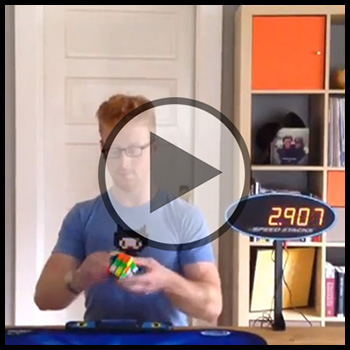## Usage

```require 'rubiks_cube'

cube = RubiksCube::Cube.new
cube.solved? #=> true
scramble = "U D B2 U B D2 B2 F' R' U2 F U' L2 F L2 B2 L2 R' U R' U' D R2 F2 B2"
cube.perform! scramble
cube.solved? #=> false
solution = RubiksCube::TwoCycleSolution.new(cube)
solution.length #=> 458
puts solution.pretty
Setup:  L2
Fix:    R U R' U' R' F R2 U' R' U' R U R' F'
Undo:   L2

Setup:  M2 D L2
Fix:    R U R' U' R' F R2 U' R' U' R U R' F'
Undo:   L2 D' M2

Setup:  U' F' U
Fix:    R U R' U' R' F R2 U' R' U' R U R' F'
Undo:   U' F U
...
```

## Setting Rubik's Cube State

If you have a scrambled Rubik's Cube sitting in front of you right now, you may not know how to get it back to the solved position. That's OK! We can set the Rubik's Cube state manually and learn how to solve it.

Starting with the F face, enter the color of each sticker from top-left to bottom-right. You may use any characters you like to describe the color of your cube, but make sure all six colors are unique. Whitespace is not important.

The cube state must be entered in the following order:

1. F: Front Face
2. R: Right Face
3. B: Back Face
4. L: Left Face
5. U: Up Face (top)
6. D: Down Face (bottom)

See the examples below for more help.

### Examples

Entering cube state manually can be confusing at first. Here are some examples to help you out.

#### Solved Cube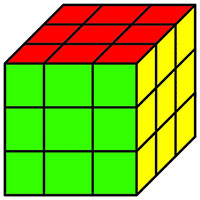A Rubik's Cube is solved by default.

```require 'rubiks_cube'

cube = RubiksCube::Cube.new
cube.state = < true
```

#### Slightly Scrambled Cube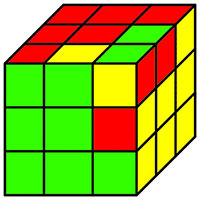Our cube is now slightly scrambled.

```require 'rubiks_cube'

cube = RubiksCube::Cube.new
cube.state = < false
```

#### Fully Scrambled Cube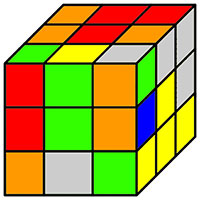Here's a fully scrambled cube. This looks hard to solve. We can start our cube in this state if we like and have the solver tell us a solution.

Here's the scramble we used to mix the cube if you'd like to try it yourself:

```U D B2 U B D2 B2 F' R' U2 F U' L2 F L2 B2 L2 R' U R' U' D R2 F2 B2
```
```require 'rubiks_cube'

cube = RubiksCube::Cube.new
cube.state = < false
```

## Turning a Rubik's Cube

Each Rubik's Cube face ( L, R, F, B, D, U ) can be turned clockwise manually by calling the appropriate method on the cube. For example, if we'd like to turn the right face twice, the down face once, and the back face three times:

```require 'rubiks_cube'

cube = RubiksCube::Cube.new
cube.r.r.d.b.b.b
```

Most people will prefer to use standard Rubik's Cube algorithm notation for turning the cube. Here's the same example with with cube notation:

```require 'rubiks_cube'

cube = RubiksCube::Cube.new
cube.perform! "R2 D B'"
```

Performing face turns on the cube changes the state.

```require 'rubiks_cube'

cube = RubiksCube::Cube.new
cube.perform!(
"U D2 F2 L' R' D' B' U' D L D U2 B' L2 F2 R' U D F2 B' R' F2 U F2 B"
)
cube.solved? #=> false
cube.state
"DB UF RB RF LB DL UR RD FD UB LF UL FDL DFR UBR BDR UFL LDB FUR LBU"
```

## Solving a Rubik's Cube

We've provided a simple two-cycle solution to help you solve the Rubik's Cube. This solution is very inefficient, but wonderful for humans. Using the two-cycle solution, you can quickly learn how to solve the Rubik's Cube without using the computer.

### Two Cycle Solution

The two-cycle solution is a popular solution used to solve the Rubik's Cube blindfolded. It requires little memorization and takes little time to learn. Solutions usually range from 400-600 turns, but most of those turns are quickly executed. We can easily achieve times of less than 4 minutes with this solution.

The two-cycle solution solves the cube by swapping two cubies at a time until all cubies are in the correct location. This is the permutation step. After the cubies are permuted, we then rotate two cubies at a time (in their current location) until all are oriented correctly. This is the orientation step. We call this the two-cycle solution because everything is done in pairs.

You can use the

`rubiks_cube`
gem to learn the two-cycle solution. For each step, we provide setup moves, the fixing algorithm (either changing permutation or orientation), and the undo moves. Pay close attention to how the cube moves and you will be solving by yourself in no time.

See Usage for an example of the

`TwoCycleSolution`

#### How It Works: Permutation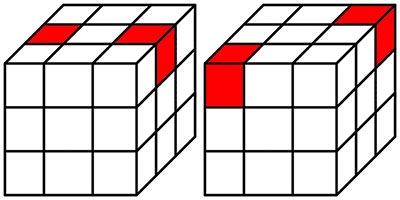The permutation step is completed using only two algorithms. One swaps two edges and the other swaps two corners. The diagram above shows which edges and corners are swapped.

When a solution is calculated, we present a 'setup' algorithm (which gets the cubies into a position where they can be swapped), then we present one of the two swapping algorithms, followed by an 'undo' algorithm that reverses the setup move.

#### How It Works: Orientation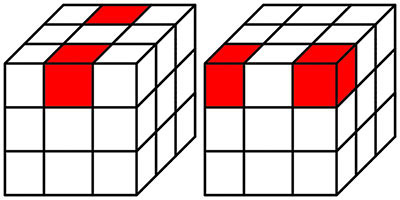The orientation step is completed using only two algorithms. One flips two edges and the other rotates two corners (one clockwise and the other counter-clockwise). The diagram above shows which edges and corners are rotated.

When a solution is calculated, we present a 'setup' algorithm (which gets the cubies into a position where they can be rotated), then we present one of the two rotation algorithms, followed by an 'undo' algorithm that reverses the setup move.

## Algorithms

All algorithms can be found in

`RubiksCube::Algorithms`

### Algorithm Notation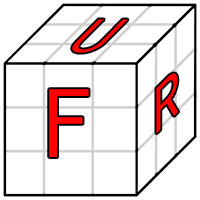Rubik's Cube algorithm notation is easy to understand, but may look confusing at first. Each face is represented by a letter:

• L: Left Face
• R: Right Face
• F: Front Face
• B: Back Face
• U: Up Face (top)
• D: Down Face (bottom)

When we see a letter in an algorithm, then we turn that face 90 degrees clockwise. To determine which direction is clockwise, rotate the cube so that you are looking at the face, then make the turn.

Faces may be followed by one of two modifiers:

• ': Rotate the face 90 degrees counter-clockwise
• 2: Rotate the face 180 degrees (two turns)

For example, if we want to apply the algorithm

`F2 B D' R`
, then we would take these steps:
1. Rotate F face 180 degrees (two turns)
2. Rotate B face 90 degrees clockwise
3. Rotate D face 90 degrees counter-clockwise
4. Rotate R face 90 degrees clockwise

#### M Slice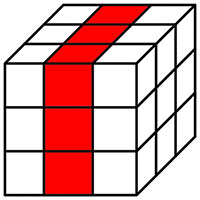There is one special algorithm notation that does not map to a face. This is called the M slice. The M slice is the middle vertical layer of the Rubik's Cube.

When you see M, then rotate this slice 90 degrees clockwise. To figure out which direction is clockwise, look at the L face.

For example, if we want to apply the algorithm

`M2 F M2`
, then we would take these steps:
1. Rotate M slice 180 degrees (two turns)
2. Rotate F face 90 degrees clockwise
3. Rotate M slice 180 degrees (two turns)

## Installation

```\$ gem install rubiks_cube
```